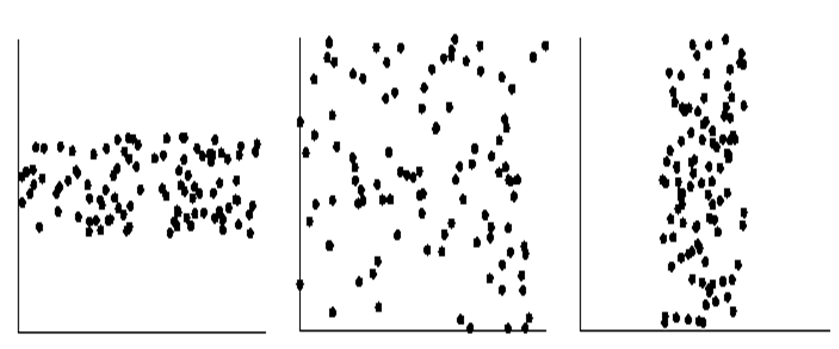# This is why you should visualize your data!

In the data science and data mining communities, several practitioners are applying various algorithms on data, without attempting to visualize the data.  This is a big mistake because sometimes, visualizing the data greatly helps to understand the data. Some phenomena are obvious when visualizing the data. In this blog post, I will give a few examples to convince you that visualization can greatly help to understand data.

An example of why using statistical measures may not be enough

The first example that I will give is a the Francis Anscombe Quartet.  It is a set of four datasets consisting of X, Y points. These four datasets are defined as follows:

#### 6.89

To get a feel of the data, the first thing that many  would do is to calculate some statistical measures such as the mean, average, variance, and standard deviation.  This allows to measure the central tendency of data and its dispersion. If we do this for the four above datasets, we obtain:

Dataset 1:   mean of X = 9, variance of X= 11, mean of Y = 7.5, variance of Y = 4.125
Dataset 2:   mean of X = 9, variance of X= 11, mean of Y = 7.5, variance of Y = 4.125
Dataset 3:   mean of X = 9, variance of X= 11, mean of Y = 7.5, variance of Y = 4.125
Dataset 4:   mean of X = 9, variance of X= 11, mean of Y = 7.5, variance of Y = 4.125

So these datasets appears quite similar. They have exactly the same values for all the above statistical measures.  How about calculating the correlation between X and Y for each dataset to see how the points are correlated?

Dataset 1:   correlation 0.816
Dataset 2:  correlation 0.816
Dataset 3:  correlation 0.816
Dataset 4:  correlation 0.816

Ok, so these datasets are very similar, isn’t it?  Let’s try something else. Let’s calculate the regression line of each dataset (this means to calculate the linear equation that would best fit the data points).

Dataset 1:  y = 3.00 + 0.500x
Dataset 2:  y = 3.00 + 0.500x
Dataset 3:  y = 3.00 + 0.500x
Dataset 4:  y = 3.00 + 0.500x

Again the same!  Should we stop here and conclude that these datasets are the same?

This would be a big mistake because actually, these four datasets are quite different! If we visualize these four datasets with a scatter plot, we obtain the following:

This shows that these datasets are actually quite different. The lesson from this example is that by visualizing the data, difference sometimes becomes quite obvious.

Visualizing the relationship between two attributes

Simple visualizations techniques like scatter plots are also very useful for quickly analyzing the relationship between pairs of attributes in a dataset. For example, by looking at the two following scatter plots, we can quickly see that the first one show a positive correlation between the X and Y axis (when values on the X axis are greater, values on the Y axis are generally also greater), while the second one shows a negative correlation (when values on the X axis are greater, values on the Y axis are generally also smaller).(a) positive correlation  (b) negative correlation (Credit: Data Mining Concepts and Techniques, Han & Kamber)

If we plot two attributes on the X and Y axis of a scatter plot and there is not correlation between the attributes, it may result in something similar to the following figures:No correlation between the X and Y axis (Credit: Data Mining Concepts and Techniques, Han & Kamber)

These examples again show that visualizing data can help to quickly understand the data.

Visualizing outliers

Visualization techniques can also be used to quickly identify outliers in the data. For example in the following chart, the data point on top can be quickly identified as an outlier (an abnormal value).

Visualizing clusters

In data mining, several clustering algorithms have been proposed to identify clusters of similar values in the data. These clusters can also often be discovered visually for low-dimensional data. For example, in the following data, it is quite apparent that there are two main clusters (groups of similar values), without applying any algorithms.

Conclusion

In this blog post, I have shown a few simple examples of how visualization can help to quickly see patterns in the data without actually applying any fancy models or performing calculations. I have also shown that statistical measures can actually be quite misleading if no visualization is done, with the classic example of the Francis Anscombe Quartet.

In this blog post, the examples are mostly done using scatter plots with 2 attributes at a time, to keep things simple. But there exists many other types of visualizations.

Philippe Fournier-Viger is a professor of Computer Science and also the founder of the open-source data mining software SPMF, offering more than 120 data mining algorithms.

(Visited 190 times, 1 visits today)

### Related posts:

1.jean marc yao POKOU on said:
2.Ananthi on said: